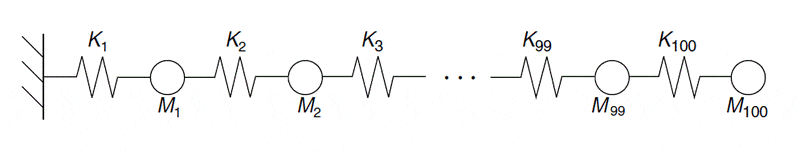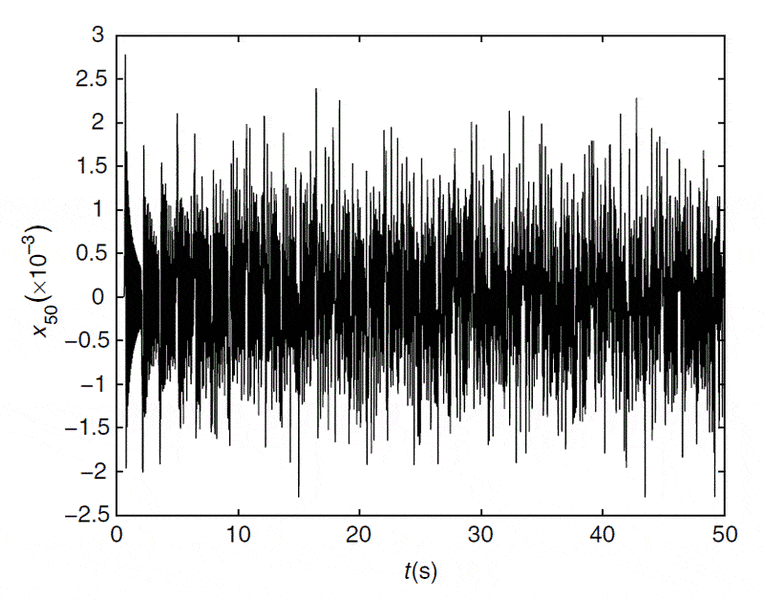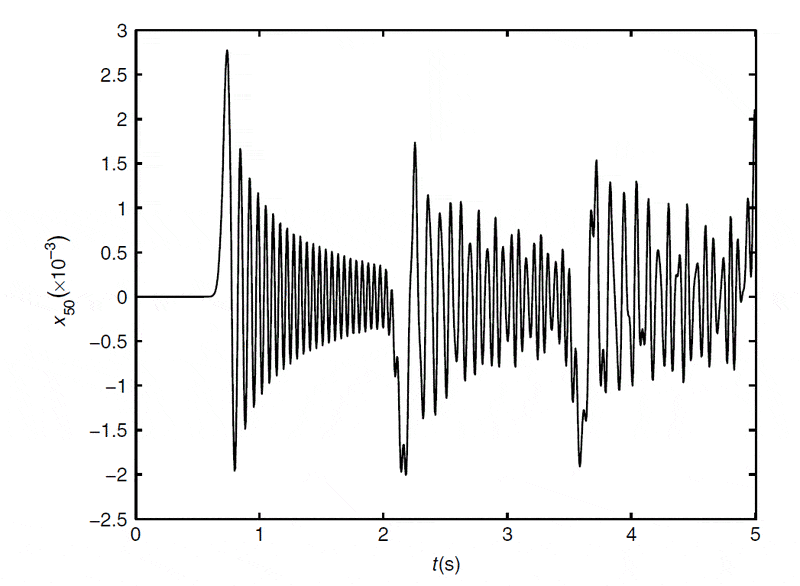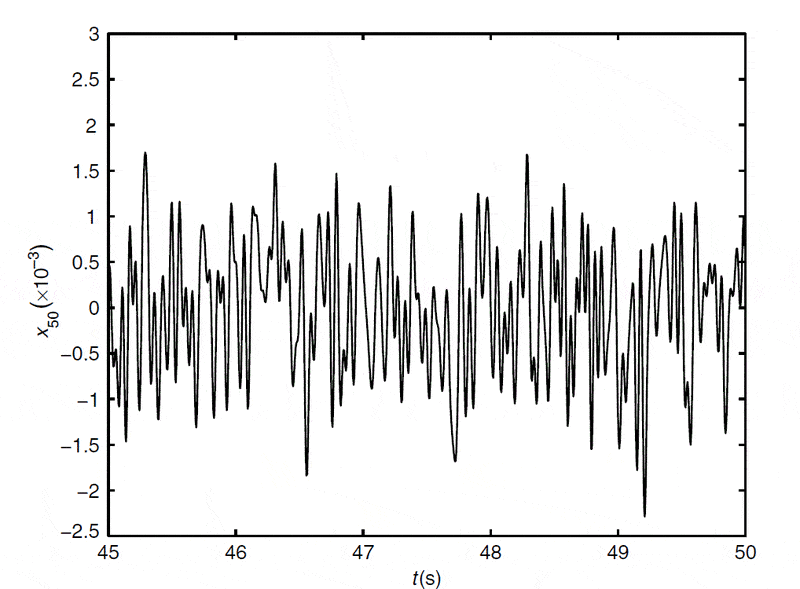# Free vibration of 100 DOF spring

Hi,

I have just started reading of "finite element model updating" which is a statistical means of updating the finite element model when there is source of uncertainty in the modelling parameters, modelling error, etc.

My question below is NOT related to finite element model updating but to a free vibration problem of linear spring with 100 degrees of freedom. I came across this problem whilst reading a text book on the subject of "Finite element model updating"

Let us consider a 100 degree of freedom system linear spring as shown in the figure below;The figure below shows the free vibration of a mass M50 located in the middle.

Note: Initial conditions - zero initial conditions except that the mass at the right end has an initial displacement of 0.01 m.The figures below zoom into the first and last 5 seconds of the response.First 5 secondsLast 5 seconds

Following points may be observed from the above figures (as stated in the text I am reading)

1) Response of first 0.6 seconds was almost 0 since the wave has not yet arrived

My question: What is the wave that the author talks about here?

2) After the first wave arrived, the respond reached maximum and decayed rapidly

My question: Again what is this first wave? It is a free vibration problem. There is no damping in the model. Why does the response decay?

3) If the response of the last 5 seconds is taken, you get random response.

What is the reason of behaviour 1,2 and 3 ?

IS it a redundant problem with insufficient information?
Is it an incorrectly formulated problem?

DrClaude
Mentor
1) Response of first 0.6 seconds was almost 0 since the wave has not yet arrived

My question: What is the wave that the author talks about here?

The compression wave. Slightly displacing the last mass creates a sound wave that will then propagate in the system.

2) After the first wave arrived, the respond reached maximum and decayed rapidly

My question: Again what is this first wave? It is a free vibration problem. There is no damping in the model. Why does the response decay?

The decay is due to the fact that wave continues to travel along the springs. The energy is spreading to the neighboring springs and masses.

3) If the response of the last 5 seconds is taken, you get random response.

What is the reason of behaviour 1,2 and 3 ?
Because of the high number of degrees of freedom, after a while you get the masses moving about in a random fashion, similar to gas particles bouncing off each other.

Nidum
Gold Member
The perturbation front . It could take a relatively long time for the disturbance caused by perturbing the RH outer mass to reach the number 50 mass .

On the first pass all springs and masses to the left of the perturbation front are undisturbed and have no motion . All those springs and masses to the right of the right of the perturbation front are disturbed and are in motion .

Nidum
Gold Member
Do the sums for a system with a manageable number of springs and masses .

Last edited:
Dear All,

Thank you very much for the answers. Can I say as below:

1) Response of first 0.6 seconds was almost 0 since the wave has not yet arrived

My question: What is the wave that the author talks about here?

That means: The wave corresponds to the disturbance / perturbation . IT take about 0.6 seconds for the disturbance to travel from the outermost mass (100th mass) to reach the 50th mass. Had it been the first mass, it would have taken more than 0.6 seconds. Am I right?

2) After the first wave arrived, the respond reached maximum and decayed rapidly

My question: Again what is this first wave? It is a free vibration problem. There is no damping in the model. Why does the response decay?

The decay is due to the fact that the perturbation / disturbance has travelled from 50th mass to innermost masses. The disturbance has been distributed. Am I right?

3)
If the response of the last 5 seconds is taken, you get random response.

Distribution of the disturbance means reaching a steady state which is (in this case) a random vibration. Am I right?

Thanks again
Kajal

Can anyone please confirm that the above (what I stated) are correct?
With affection,
Kajal

DrClaude
Mentor
That means: The wave corresponds to the disturbance / perturbation . IT take about 0.6 seconds for the disturbance to travel from the outermost mass (100th mass) to reach the 50th mass. Had it been the first mass, it would have taken more than 0.6 seconds. Am I right?

Correct. You can use the observation to figure out the speed of sound in the system.

The decay is due to the fact that the perturbation / disturbance has travelled from 50th mass to innermost masses. The disturbance has been distributed. Am I right?

Initially, it is not yet distributed, but mostly just passes along to the innermost masses. This is why it looks exactly like a damped oscillation. Note that the amplitude of the oscillation goes back up as the wave bounces back from the wall. In time, the perturbation gets distributed over all masses.

Distribution of the disturbance means reaching a steady state which is (in this case) a random vibration. Am I right?

I would say that the system reaches an internal equilibrium.

Nidum
Gold Member
Because of the high number of degrees of freedom, after a while you get the masses moving about in a random fashion, similar to gas particles bouncing off each other.

I've watched this happen in a vibration lab . Just a small number of springs and masses connected in series . Odd thing though is that the motion did not always transition slowly from systematic to chaotic . In some experiments the motion flipped from systematic to chaotic almost instantly .

Really though using a simple axial force/displacement model for systems where there are more than a few springs and masses is not very realistic . There are other things going on which could modify the motion considerably

DrClaude
Mentor
I've watched this happen in a vibration lab . Just a small number of springs and masses connected in series . Odd thing though is that the motion did not always transition slowly from systematic to chaotic . In some experiments the motion flipped from systematic to chaotic almost instantly .
Could it be when the springs can longer be considered linear?

Nidum
Gold Member
The large motion disturbance wave moves along the system at a rate determined by the dynamic characteristics of the springs and masses .

The speed of sound in the metal of the springs determines the maximum speed at which the associated stress wave can propagate .

Could it be when the springs can longer be considered linear?

Almost certainly due to effects of imperfections in the springs causing non linearity and off axis secondary motions . There is always going to be some damping as well but effect of that is difficult to estimate .

Dear All,

1) Do you think that this problem is unrealistic? That is, not a 'practical problem'?

Do you think the problem is not completely defined? That is, for it to be completely defined, initial states of more degrees of freedom should have been provided than just the outermost degree of freedom?

2)
Correct. You can use the observation to figure out the speed of sound in the system

I've heard this before, that is, using the observation to figure out the speed of sound in the system. I do not physically understand that how is this related to 'speed of sound'

With regards and affection,
kajal

DrClaude
Mentor
1) Do you think that this problem is unrealistic? That is, not a 'practical problem'?

Do you think the problem is not completely defined? That is, for it to be completely defined, initial states of more degrees of freedom should have been provided than just the outermost degree of freedom?
To the contrary, I think this is a very interesting problem. It allows to understand some fundamental things about wave propagation. And the system is completely defined, since all springs are at their equilibrium position, except one.

I've heard this before, that is, using the observation to figure out the speed of sound in the system. I do not physically understand that how is this related to 'speed of sound'
The speed of sound is the same as the speed of propagation of the perturbation. Sound is simply a propagating compression wave.

Nidum